编程技术资讯
 程序员段子
 程序人生
 秒杀专栏
 名企资讯
 运维监控
 悟空教程-Java
 悟空教程-Python
 悟空教程-大数据
 人事面试
 技术面试
 大禹编程
 SpringBoot
 SpringCloud(F版)
 SpringCloud(D版)
 spring
 Docker
 Dubbo
 IntelliJ IDEA
 Linux
 Nginx
 Tomcat
 Quartz
 微信小程序
 阿里云
 Java
 Python
 Java案例
 小程序源码
 Java源码
 手机最新资讯
 Sql Server
 MySQL
 MongoDB
 免费教程
 前端开发
 node.js
 CSS
 移动端
 jQuery
 Sublime Test
 JavaScript
 HTML
 Vue.js
 HTTPS
 分布式事物
 Redis
 JSON

# 优化过程 PK ：Julia 能打败 Python 和 R 笑到最后吗？

26

R9 年
Julia6 个月
Python新手

Julia 布道者 ChrisRackauckas 曾经说过：

### 优化问题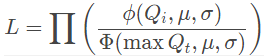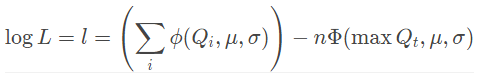### Julia 的测试情况

Julia 第一次优化

Julia 在执行第一次优化用了 7 秒，比 R 和 Python 都慢。对此，ChrisRackauckas 指出：

using Distributions, Optim# hard coded data\observationsodr=[0.10,0.20,0.15,0.22,0.15,0.10,0.08,0.09,0.12]
Q_t = quantile.(Normal(0,1), odr)# return a function that accepts [mu, sigma] as parameterfunction neglik_tn(Q_t)
maxx = maximum(Q_t)
f(μσ) = -sum(logpdf.(Truncated(Normal(μσ,μσ), -Inf, maxx), Q_t))
f
end

neglikfn = neglik_tn(Q_t)# optimize!# start searching @time res = optimize(neglikfn, [mean(Q_t), std(Q_t)]) # 7.5 seconds@time res = optimize(neglikfn, [mean(Q_t), std(Q_t)]) # 0.000137 seconds# the \mu and \sigma estimatesOptim.minimizer(res) # [-1.0733250637041452,0.2537450497038758] # or# use fieldnames(res) to see the list of field names that can be referenced via . (dot)res.minimizer # [-1.0733250637041452,0.2537450497038758]

Results of Optimization Algorithm
* Starting Point: [-1.1300664159893685,0.22269345618402703]
* Minimizer: [-1.0733250637041452,0.2537450497038758]
* Minimum: -1.893080e+00
* Iterations: 28
* Convergence: true
*  √(Σ(yᵢ-ȳ)²)/n < 1.0e-08: true
* Reached Maximum Number of Iterations: false
* Objective Calls: 59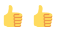Truncated(DN, lower, upper) 是定义截断分布的非常简单的方法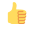logpdf 函数适用于任何分布式函数输出结果条理清晰，可读性强

Julia 的不足：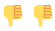如果只是处理 10 秒内的简单问题，7.5 秒的编译时间会很烦人

### R 的测试情况

R 有一个 truncnorm 用于处理截断正态

odr=c(0.10,0.20,0.15,0.22,0.15,0.10,0.08,0.09,0.12)
x = qnorm(odr)

library(truncnorm)
neglik_tn = function(x) {
maxx = max(x)
resfn = function(musigma) {
-sum(log(dtruncnorm(x, a = -Inf, b= maxx, musigma, musigma)))
}

resfn
}

neglikfn = neglik_tn(x)

system.time(res <- optim(c(mean(x), sd(x)), neglikfn))
res

$par  -1.0733354 0.2537339$value
 -1.89308

$counts function gradient 55 NA$convergence
 0

\$message
NULL

R 的优势：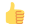又处理截断正态的专用包马上输出结果，编译比 Julia 快

R 的不足：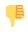截断正态没有对数密度; 没有简单的方法来定义任意分布的截断分布; 稀疏输出

### Python 的测试情况

import numpy as npfrom scipy.optimize import minimizefrom scipy.stats import norm# generate the dataodr=[0.10,0.20,0.15,0.22,0.15,0.10,0.08,0.09,0.12]
Q_t = norm.ppf(odr)
maxQ_t = max(Q_t)# define a function that will return a return to optimize based on the input datadef neglik_trunc_tn(Q_t):
maxQ_t = max(Q_t)    def neglik_trunc_fn(musigma):
return -sum(norm.logpdf(Q_t, musigma, musigma)) + len(Q_t)*norm.logcdf(maxQ_t, musigma, musigma)    return neglik_trunc_fn# the likelihood function to optimizeneglik = neglik_trunc_tn(Q_t)# optimize!res = minimize(neglik, [np.mean(Q_t), np.std(Q_t)])
res

      fun: -1.8930804441641282
hess_inv: array([[ 0.01759589,  0.00818596],
[ 0.00818596,  0.00937868]])
jac: array([ -3.87430191e-07,   3.33786011e-06])
message: 'Optimization terminated successfully.'
nfev: 40
nit: 6
njev: 10
status: 0
success: True
x: array([-1.07334252,  0.25373624])

Python 的优势：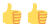易于学习，各种支持非常好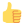能很快输出结果，比 Julia 编译快

Python 的不足：输出的可读性有待提高

Julia

R易于使用可读性对比 Julia 较差
Python易于使用可读性对比 Julia 较差近日，在美国推出满一年的Facebook视频服务Watch，宣布正式向全球推广，这预示着视频领域中，YouTube...据彭博社北京时间9月19日报道，科技行业最引人注目的法律大战可能正在进入尾声。据高通CEO史蒂夫·莫伦科夫（Ste...

Java帮帮公众号生态

Java帮帮公众号生态

 Java帮帮-微信公众号Java帮帮-微信公众号将分享做到极致 Python帮帮-公众号Python帮帮-公众号人工智能,爬虫,学习教程 大数据驿站-微信公众号大数据驿站-微信公众号一起在数据中成长 程序员服务区-公众号程序员服务区-公众号吃喝玩乐，听学吐画
Java帮帮学习群生态

Java帮帮学习群生态

Java帮帮生态承诺

Java帮帮生态承诺